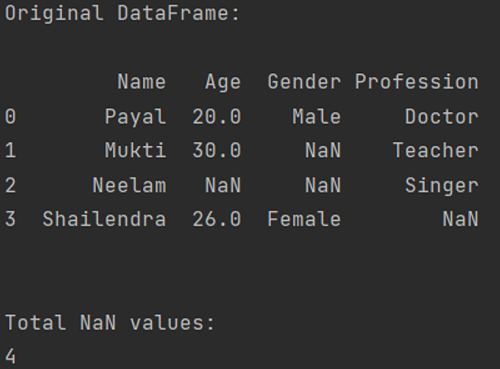# Check for NaN Values in Pandas DataFrame

In this tutorial, we will learn how to check for the NaN values in a pandas DataFrame? By Pranit Sharma Last updated : April 19, 2023

## Checking If Any Value is NaN in a Pandas DataFrame

To check for NaN values in pandas DataFrame, simply use the DataFrame.isnull().sum().sum(). Here, the isnull() returns a True or False value. Where, True means that there is some missing data and False means that the data is not null and the sum() returns the count of (True) NaN values generated from the isnull() method.

Let us understand with the help of an example.

## Python Code for Check for NaN Values in Pandas DataFrame

```# Importing pandas package
import pandas as pd

# To create NaN values, you must import numpy package,
# then you will use numpy.NaN to create NaN values
import numpy as np

# Creating a dictionary with some NaN values
d = {
"Name":['Payal','Mukti','Neelam','Shailendra'],
"Age":[20,30,np.NaN,26],
"Gender":['Male',np.NaN,np.NaN,'Female'],
"Profession":['Doctor','Teacher','Singer',np.NaN]
}

# Now, Create DataFrame
df=pd.DataFrame(d)

# Printing the original DataFrame
print("Original DataFrame:\n")
print(df,"\n\n")

# Counting NaN values
NAN=df.isnull().sum().sum()

# Now, Printing the Number of NaN values
print("Total NaN values:")
print(NAN)
```

### Output